Courses

Filter Design by Impulse Invariance & Bilinear Transformation Notes | Study Digital Signal Processing - Electrical Engineering (EE)

Electrical Engineering (EE): Filter Design by Impulse Invariance & Bilinear Transformation Notes | Study Digital Signal Processing - Electrical Engineering (EE)

The document Filter Design by Impulse Invariance & Bilinear Transformation Notes | Study Digital Signal Processing - Electrical Engineering (EE) is a part of the Electrical Engineering (EE) Course Digital Signal Processing.
All you need of Electrical Engineering (EE) at this link: Electrical Engineering (EE)

Filter design by impulse invariance

In the impulse variance design procedure the impulse response of the impulse response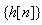of the discrete time system is proportional to equally spaced samples of the continues time filter, i.e.,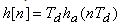where Td represents a sampling interval, since the specifications of the filter are given in discrete time domain, it turns out that Td has no role to play in design of the filter. From the sampling theorem we know that the frequency response of the discrete time filter is given by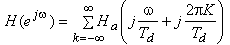Since any practical continuous time filter is not strictly bandlimited there issome aliasing. However, if the continuous time filter approaches zero at high frequencies, the aliasing may be negligible. Then the frequency response of the discrete time filter is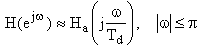We first convert digital filter specifications to continuous time filter specifications. Neglecting aliasing, we get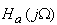specification by applying the relation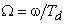(9.2)

whereis transferred to the designed filter H(z), we again use equation (9.2) and the parameter Tdcancels out.

Let us assume that the poles of the continuous time filter are simple, then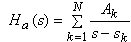The corresponding impulse response is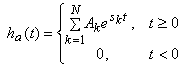Then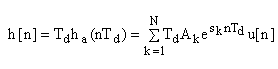The system function for this is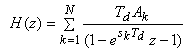We see that a pole at  s= sk in the s-plane is transformed to a pole at z = e sk Td in the z-plane. If the continuous time filter is stable, that is Re {sk} < 0 then the magnitude of eskTd will be less than 1, so the pole will be inside unit circle. Thus the causal discrete time filter is stable. The mapping of zeros is not so straight forward.

Example:

Design a lowpass IIR digital filter H(z) with maximally flat magnitude characteristics. The passband edge frequency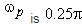with a passband ripple not exceeding 0.5dB. The minimum stopband attenuation at the stopband edge frequency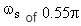is 15 dB.

We assume that no aliasing occurs. Taking Td = 1 , the analog filter has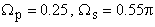, the passband ripple is 0.5dB, and minimum stopped attenuation is 15dB. For maximally flat frequency response we choose Butterworth filter characteristics. From passband ripple of 0.5 dB we get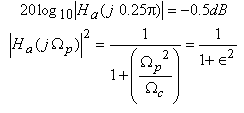at passband edge.

From this we get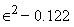From minimum stopband attenuation of 15 dB we get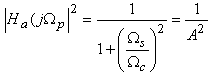at stopped edge A2 = 31.62

The inverse discrimination ratio is given by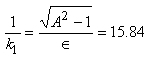and inverse transition ratio 1/k is given bySince must be integer we get N=4. By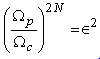we get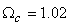The normalized Butterworth transfer function of order 4 is given by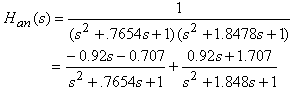This is for normalized frequency of 1 rad/s. Replace by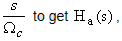from this we get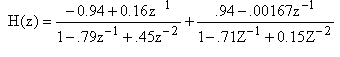Bilinear Transformation

This technique avoids the problem of aliasing by mapping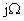axis in the s-plane to one revaluation of the unit circle in the z-plane.

If  Ha(s) is the continues time transfer function the discrete time transfer function is detained by replacing with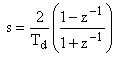(9.3)

Rearranging terms in equation (9.3) we obtain.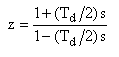Substituting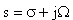, we get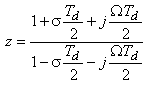If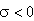, it is then magnitude of the real part in denominator is more than that of the numerator and so. Similarly if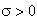, than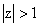for all. Thus poles in the left half of the s-plane will get mapped to the poles inside the unit circle in z-plane. If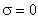then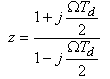So,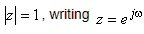we getrearranging we get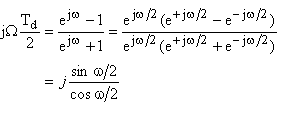or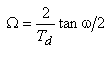(9.5)
or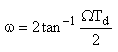(9.6)

The compression of frequency axis represented by (9.5) is nonlinear. This is illustrated in figure 9.4.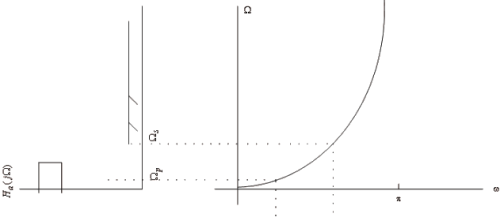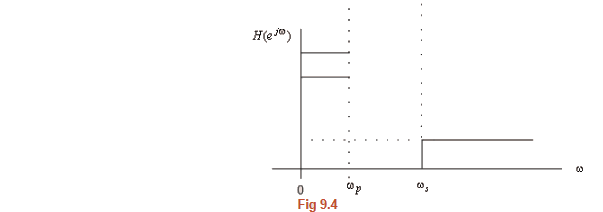Because of the nonlinear compression of the frequency axis, there is considerable phase distortion in the bilinear transformation.

Example

We use the specifications given in the previous example. Using equation (9.5) with Td = 2 we get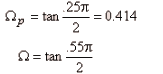The document Filter Design by Impulse Invariance & Bilinear Transformation Notes | Study Digital Signal Processing - Electrical Engineering (EE) is a part of the Electrical Engineering (EE) Course Digital Signal Processing.
All you need of Electrical Engineering (EE) at this link: Electrical Engineering (EE)Use Code STAYHOME200 and get INR 200 additional OFF Use Coupon Code

Top Courses for Electrical Engineering (EE)Digital Signal Processing

3 videos|50 docs|54 tests

Top Courses for Electrical Engineering (EE)Track your progress, build streaks, highlight & save important lessons and more!

,

,

,

,

,

,

,

,

,

,

,

,

,

,

,

,

,

,

,

,

,

;